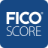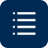# Compound Interest Explained

You're probably familiar with the interest rate a bank pays you when you put money in a savings account, for example. Compound interest takes saving to another level, because they represent interest you earn on both the money you put in and the interest on that money. This interest on top of interest can quickly build upon what you save.

## What is Compound Interest?

Unlike simple interest, where you earn a rate of interest annually without compounding, compound interest allows interest to grow on top of interest. Their interest is calculated based on the initial principal amount (the initial size of the deposit) as well as the interest it accumulates over time.

Compound interest is essential to understand when it comes to savings. Savings products like conventional savings accounts, money market accounts, CDs, and retirement accounts all use compound interest to grow their deposited funds.

## How Does Compound Interest Work?

Compound interest works by adding accumulated interest over time to your principal. This means that accounts that compound interest grow more rapidly compared to simple interest without compounding.

## What is the formula used for compound interest?

The compound interest formula considers:

• Interest rate
• Time and compounding periods, which are typically daily, monthly or annually

The result? The amount of interest you'll save over that period of time.

The compound interest formula is:

Initial principal amount multiplied by (1 plus the annual interest rate, divided by the number of compounding periods in a year, raised to the number of compounding periods multiplied by time)

OR

A = P (1 + r/n) (nt)
P is your principal (initial deposit)
r is the annual rate of interest as a decimal
t is the length of time the principal is on deposit
n is the number of times interest is compounded per unit of t
A is the future value you will have at the end of the time period

You can use the formula to explore different scenarios and see how compound interest can make a real difference in your savings and life goals.

## Compound interest formula in action: daily, monthly and yearly compounding

### Annual interest compounding examples

Let's see how the compounding interest formula works to boost your savings. For example, say you want to deposit \$10,000 in a savings account that earns a 2.3%* annual rate of return. Use the compound interest formula to calculate the amount you would have at the end of a savings period for different compounding options.

A = P (1 + r/n) (nt)

Starting with a balance of \$10,000 and a 2.3%* annual rate of return, after one year you can possibly end up with as much as \$10,233 in a savings account.

 Formula Compounding Yearly Compounding Monthly Compounding Daily Principal P \$10,000 \$10,000 \$10,000 Rate r .023* .023* .023* Time t 1 1 1 Periods n 1 12 365 Future Value A \$10,230 \$10,232 \$10,233

Notice how the more frequently your interest is compounded, the more interest you earn.

What could happen if you save that \$10,000 longer - say 5 years instead? That initial deposit can grow via compounding by more than \$1,200 with a 2.3%* annual rate.

 Formula Compounding Yearly Compounding Monthly Compounding Daily Principal P \$10,000 \$10,000 \$10,000 Rate r .023* .023* .023* Time t 5 5 5 Periods n 1 12 365 Future Value A \$11,204 \$11,217 \$11,219

If you really want your savings to reap the advantages of compound interest, consider the potential impact of additional regular contributions. For example, by contributing \$100 per month to your savings, it may grow to as much as \$17,565 after 5 years at a 2.3%* annual rate of return.

 Formula Compounding Yearly Compounding Monthly Compounding Daily Principal P \$10,000 \$10,000 \$10,000 Rate r .023* .023* .023* Time t 5 5 5 Periods n 1 12 365 Monthly Deposit \$100 \$100 \$100 Future Value A \$17,565 \$17,582 \$17,583

Remember, you started with a balance of simply \$10,000.

## How Does Compound Interest Grow Savings Over Time?

Compound interest grows savings over time by applying an interest rate to a continuously growing principal. With this formula in place, a principal amount will grow faster as the principal becomes larger. This acceleration continues as long as the compounding continues to take place.

Compounding interest is a great option for anyone looking to grow a savings account or investment. The nature of the compound interest formula means that as the account grows, it will pick up momentum and increase at a larger scale.

## What is a Compounded Annual Growth Rate (CAGR)?

The Compounded Annual Growth Rate is a type of compound interest calculation that tries to estimate an ideal rate of return for an investment, provided that all the profits of an investment are put back into the investment at a regular period. The CAGR sees frequent usage in the trading of stock options, investment portfolios, and other securities, where it's used to calculate the annual growth rate of stocks that are experiencing volatility.

### What you should know about compound interest and your savings accounts

When you open or add money to a savings account, read the details to determine how frequently interest compounds for your account. You can also use a compound interest calculator to estimate how much money you are likely making by earning interest on your interest.

Compound interest can result in you having more money in your account each month. You can have more financial freedom and an easier journey towards your savings goals.

Looking to save money for your first house?

Hoping to plan a vacation to remember?

Compound interest can help you achieve your goals faster, increase your savings power and set you on a strong financial path. No matter what you're saving for, you are helping out your future self in a measurable way by using compound interest to increase your savings.

*Rate provided is for illustrative purposes only.

•••